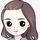cancel
Showing results for
Did you mean:Helper I

## How to optimize my dax to make it faster

Hi All

Dax:

_Actuals_MTD_D =

VAR ST =SUM(wt_fin_pl_actuals_monthly_summary[total_actual_Monthly_values])

VAR CurrentItem = SELECTEDVALUE( 'wt_fin_staging_pl_accounts_mapping_sorting'[Row_Index] )

VAR _1 = CALCULATE(ST,wt_fin_staging_pl_accounts_mapping_sorting[Level 1] IN { "AC=10000" })
VAR _2 =CALCULATE( ST, wt_fin_staging_pl_accounts_mapping_sorting[Level 1] IN { "AC=10900" } )
VAR _3 = [_NetSales_NoDrill]
VAR _5 =[_StaffSalary_NoDrill]
VAR _6 =[_CFT_NoDrill]
VAR _7 =[_ICTS_NoDrill]
VAR _8 =[_SFLICs_NoDrill]
VAR _9 = [_HP_NoDrill]
VAR _10 =[_Sevarnces_NoDrill]
VAR _11 = [_OtherStaffCost_NoDrill]
VAR _12 = [_SCPreB_NoDrill]
VAR _16 = [Establishment_MTD]
VAR _19 = [_PC_NoDrill]
VAR _20 = [_CexNWWCAP_NoDrill]
VAR _21 = [_OC_NoDrill]
VAR _22 = [_ITC_NoDrill]
VAR _23 = [_FGC_NoDrill]
VAR _24 = [_OA_NoDrill]
VAR _25 = [_G&Cost_NoDrill]
VAR _28 = CALCULATE(ST,wt_fin_staging_pl_accounts_mapping_sorting[Level 2] IN { "AC=17115", "AC=17120", "AC=17125", "AC=17320" } )
VAR _31 = [_PBOP_NoDrill]
VAR _34 = [_CE_NoDrill]
VAR _35 = [_S&PSA_NoDrill]
VAR _36 = [_OtherIncentive_NoDrill]
VAR _37 = [Incentives_MTD]
VAR _40 = [_OP_NoDrill]
VAR _43 = [_E&OI_NoDrill]
VAR _45 = [_PBIT_NoDrill]
VAR _48 = [_ClossingHC_NoDrill]
VAR _49 = [_ClossingFreelance_NoDrill]

---------------------------Accounts----------------------------

VAR R1 =1-- min('wt_fin_staging_pl_accounts_mapping_sorting'[Account_Summary]) = "Billings"
VAR R2 = 2--min('wt_fin_staging_pl_accounts_mapping_sorting'[Account_Summary]) = "Revenue"
VAR R3 =3--min('wt_fin_staging_pl_accounts_mapping_sorting'[Account_Summary]) = "Net Sales"
VAR R5 = 5--min('wt_fin_staging_pl_accounts_mapping_sorting'[Account_Summary]) = "        Staff salaries"
VAR R6 = 6--min('wt_fin_staging_pl_accounts_mapping_sorting'[Account_Summary]) = "        Consultants/freelances/temps"
VAR R7 = 7--min('wt_fin_staging_pl_accounts_mapping_sorting'[Account_Summary]) = "        Inter-company time of staff"
VAR R8 = 8--min('wt_fin_staging_pl_accounts_mapping_sorting'[Account_Summary]) = "    SFLICs"
VAR R9 = 9--min('wt_fin_staging_pl_accounts_mapping_sorting'[Account_Summary]) = "    Holiday Pay"
VAR R10 = 10--min('wt_fin_staging_pl_accounts_mapping_sorting'[Account_Summary]) = "    Severances"
VAR R11 = 11--min('wt_fin_staging_pl_accounts_mapping_sorting'[Account_Summary]) = "    Other staff costs"
VAR R12 = 12--min('wt_fin_staging_pl_accounts_mapping_sorting'[Account_Summary]) = "Staff costs (pre-bonus)"
VAR R16 = 16--min('wt_fin_staging_pl_accounts_mapping_sorting'[Account_Summary]) = "Establishment"
VAR R19 = 19--min('wt_fin_staging_pl_accounts_mapping_sorting'[Account_Summary]) = "    Personal costs"
VAR R20 = 20--min('wt_fin_staging_pl_accounts_mapping_sorting'[Account_Summary]) = "    Commercial ex NWC Aging Provisions"
VAR R21 = 21--min('wt_fin_staging_pl_accounts_mapping_sorting'[Account_Summary]) = "    Office costs"
VAR R22 = 22--min('wt_fin_staging_pl_accounts_mapping_sorting'[Account_Summary]) = "    IT costs"
VAR R23 = 23--min('wt_fin_staging_pl_accounts_mapping_sorting'[Account_Summary]) = "    Finance & General costs"
VAR R24 = 24--min('wt_fin_staging_pl_accounts_mapping_sorting'[Account_Summary]) = "    Overhead allocation"
VAR R25 = 25--min('wt_fin_staging_pl_accounts_mapping_sorting'[Account_Summary]) = "G&A costs"
VAR R28 = 28--min('wt_fin_staging_pl_accounts_mapping_sorting'[Account_Summary]) = "NWC Aging Provisions"
VAR R31 = 31--min('wt_fin_staging_pl_accounts_mapping_sorting'[Account_Summary]) = "PBOP"
VAR R34 = 34--min('wt_fin_staging_pl_accounts_mapping_sorting'[Account_Summary]) = "    Contractual/Earnout"
VAR R35 = 35--min('wt_fin_staging_pl_accounts_mapping_sorting'[Account_Summary]) = "    STIP & PSA"
VAR R36 = 36--min('wt_fin_staging_pl_accounts_mapping_sorting'[Account_Summary]) = "    Other Incentives"
VAR R37 = 37--min('wt_fin_staging_pl_accounts_mapping_sorting'[Account_Summary]) = "Incentives"
VAR R40 = 40--min('wt_fin_staging_pl_accounts_mapping_sorting'[Account_Summary]) = "OP"
VAR R43 = 43--min('wt_fin_staging_pl_accounts_mapping_sorting'[Account_Summary]) = "Equity and Other Income"
VAR R45 = 45--min('wt_fin_staging_pl_accounts_mapping_sorting'[Account_Summary]) = "PBIT"
VAR R48 = 48--min('wt_fin_staging_pl_accounts_mapping_sorting'[Account_Summary]) = "Closing Headcount"
VAR R49 = 49--min('wt_fin_staging_pl_accounts_mapping_sorting'[Account_Summary]) = "Closing Freelance"

RETURN
SWITCH(
CurrentItem,
1, IF(R1, _1),
2, IF(R2, _2),
3, IF(R3, _3),
5, IF(R5, _5),
6, IF(R6, _6),
7, IF(R7, _7),
8, IF(R8, _8),
9, IF(R9, _9),
10, IF(R10, _10),
11, IF(R11, _11),
12, IF(R12, _12),
16, IF(R16, _16),
19, IF(R19, _19),
20, IF(R20, _20),
21, IF(R21, _21),
22, IF(R22, _22),
23, IF(R23, _23),
24, IF(R24, _24),
25, IF(R25, _25),
28, IF(R28, _28),
31, IF(R31, _31),
34, IF(R34, _34),
35, IF(R35, _35),
36, IF(R36, _36),
37, IF(R37, _37),
40, IF(R40, _40),
43, IF(R43, _43),
45, IF(R45, _45),
48, IF(R48, _48),
49, IF(R49, _49)

)

Dax:
Reporting Cycle_DT =
VAR CurrentItem =SELECTEDVALUE( 'SelectedV_PeriodType'[ID] )
VAR _1=[_ReportingCycle_Formated_M_D]
VAR _2=[_ReportingCycle_Formated_FY_D]
VAR _3=[_ReportingCycle_Formated_YTD_D]

RETURN
SWITCH(
TRUE( ),
CurrentItem = 1,_1,
CurrentItem = 2,_2,
CurrentItem = 3,_3
)
3 REPLIES 3Helper I

Hi ,

It will be great if you provide optimize dax as per above query it will be helpful to understand thatCommunity Support

Improve Power BI Performance by Optimizing your DAX

How to make your Power BI reports faster:To optimize DAX queries

It's a little difficult to give you a suitable solution without some sample data and backend scenario(function). Could you please provide some sample in the involved tables('wt_fin_staging_pl_accounts_mapping_sorting' and 'wt_fin_staging_pl_accounts_mapping_sorting') and the functions which your above formula want to achieve? It is better if you can provide some special example to explain your expected result. Thank you.

Best Regards

Community Support Team _ Rena
If this post helps, then please consider Accept it as the solution to help the other members find it more quickly.Super User

@aamirkhan_WT , Try calculation groups or field parameters.

Calculation Groups- Measure Slicer, Measure Header Grouping, Measure to dimension conversion. Complex Table display : https://youtu.be/qMNv67P8Go0

Power BI Field Parameters — A Quick way for Dynamic Visuals: https://amitchandak.medium.com/power-bi-field-parameters-a-quick-way-for-dynamic-visuals-fc4095ae9af...

I am really not sure, why need so many formulae here.Announcements#### Exclusive opportunity for Women!

Join us for a free, hands-on Microsoft workshop led by women trainers for women where you will learn how to build a Dashboard in a Day!#### Power Platform Conference-Power BI and Fabric Sessions

Join us Oct 1 - 6 in Las Vegas for the Microsoft Power Platform Conference.Top Solution Authors
Top Kudoed Authors
Users online (4,437)